# 9s complement and 10s complement | Subtraction

Before knowing about 9’s complement and 10’s complement we should know why they are used and why their concept came into existence. The complements are used to make the arithmetic operations in digital system easier. In this article we will discuss about the following topics

1. 9’s complement
2. 10’s complement
3. 9’s complement subtraction
4. 10’s complement subtraction

Now first of all let us know what 9’s complement is and how it is done. To obtain the 9’s complement of any number we have to subtract the number with (10n – 1) where n = number of digits in the number, or in a simpler manner we have to divide each digit of the given decimal number with 9. The table given below will explain the 9’s complement more easily.

 Decimal digit 9s complement 0 9 1 8 2 7 3 6 4 5 5 4 6 3 7 2 8 1 9 0

Now coming to 10’s complement, it is relatively easy to find out the 10’s complement after finding out the 9’s complement of that number. We have to add 1 with the 9’s complement of any number to obtain the desired 10’s complement of that number. Or if we want to find out the 10’s complement directly, we can do it by following the following formula, (10n – number), where n = number of digits in the number. An example is given below to illustrate the concept of obtaining 10’s complement.
Let us take a decimal number 456, 9’s complement of this number will be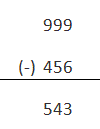10’s complement of this no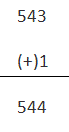9’s complement subtraction
We will understand this method of subtraction via an example
A = 215
B = 155
We want to find out A-B by 9’s complement subtraction method

First we have to find out 9’s complement of B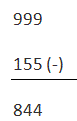Now we have to add 9’s complement of B to A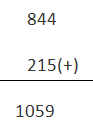The left most bit of the result is called carry and is added back to the part of the result without it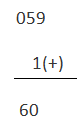Another different type of example is given
A = 4567
B = 1234
We need to find out A – B
9’s complement of B
8765
Adding 9’s complement of B with A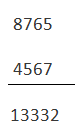Adding the carry with the result we get
3333
Now the answer is – 3333
NB: If there is no carry the answer will be – (9’s complement of the answer)

Subtraction by 10’s complement
Again we will show the procedure by an example
Taking the same data
A = 215
B = 155
10’s complement of B = 845
Adding 10’s complement of B to A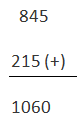In this case the carry is omitted
Taking the other example
A = 4567
B = 1234
10’s complement of B = 8766
Adding 10’s complement of B with A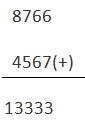To get the answer the carry is ignored
So, the answer is – 3333
NB: If there is a carry then the answer is – (10’s complement of the sum obtained)

Want To Learn Faster? 🎓
Get electrical articles delivered to your inbox every week.
No credit card required—it’s 100% free.